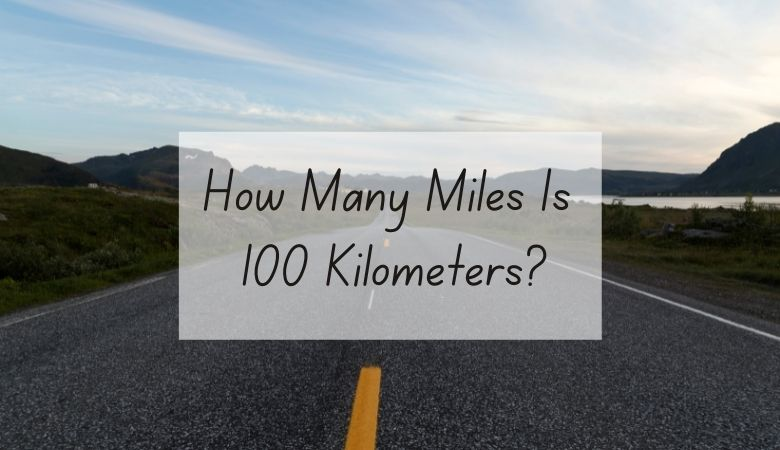# How Many Miles Is 100 Kilometers?

100 kilometers equals 62.15 miles.

Miles and kilometers are both used to measure long distances, and understanding them can make driving simpler.

However, because they’re units of measurement in different measuring systems, converting between the two can sometimes seem tricky.

Luckily, a bit of math can give you a good idea of how many miles are in 100 kilometers.

One kilometer equals about .62 miles. This means that, in order to figure out how many miles are in a given number of kilometers, you would need to divide the number of kilometers by 1.609.

In order to figure out how many miles are in 100 kilometers, you would divide 100 by 1.609.

The answer, which is about 62.15, tells you that there are just over 62 miles in 100 kilometers.

To reverse the equation and find out how many kilometers are in a certain number of miles, you would multiply the number of miles by 1.609.

For example, if you want to know how many kilometers are in 50 miles, you need to multiply 50 by 1.609.

The answer, which is about 80.45, tells you that there are about 80 and a half kilometers in 50 miles.

If you don’t feel like doing the math, use our online conversion calculator to convert different units of measurements and quickly give you the answer.

## Kilometers to miles conversion calculator

You can also use the following table to convert kilometers into miles.

## Miles and kilometers units of measurements

Miles are part of the imperial system, which is widely used in the United States. This means that distances between places in the US or on US maps are most commonly measured in miles.

Kilometers, on the other hand, are a unit of distance used in the metric system, which is common in Canada, Europe, and many other parts of the world.